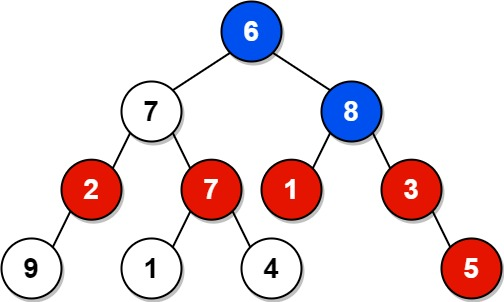# 1315. Sum of Nodes with Even-Valued Grandparent

Medium
Given the `root` of a binary tree, return the sum of values of nodes with an even-valued grandparent. If there are no nodes with an even-valued grandparent, return `0`.
A grandparent of a node is the parent of its parent if it exists.
Example 1:Input: root = [6,7,8,2,7,1,3,9,null,1,4,null,null,null,5]
Output: 18
Explanation: The red nodes are the nodes with even-value grandparent while the blue nodes are the even-value grandparents.
Example 2:Input: root = 
Output: 0
Constraints:
• The number of nodes in the tree is in the range `[1, 10^4]`.
• `1 <= Node.val <= 100`

### 解題

/**
* Definition for a binary tree node.
* type TreeNode struct {
* Val int
* Left *TreeNode
* Right *TreeNode
* }
*/
func sumEvenGrandparent(root *TreeNode) int {
ans := 0
var helper func(*TreeNode, *TreeNode, *TreeNode)
helper = func(node *TreeNode, parent *TreeNode, grandParent *TreeNode) {
if node == nil {
return
}
if grandParent != nil && grandParent.Val % 2 == 0 {
ans += node.Val
}
helper(node.Left, node, parent)
helper(node.Right, node, parent)
}
helper(root, nil, nil)
return ans
}